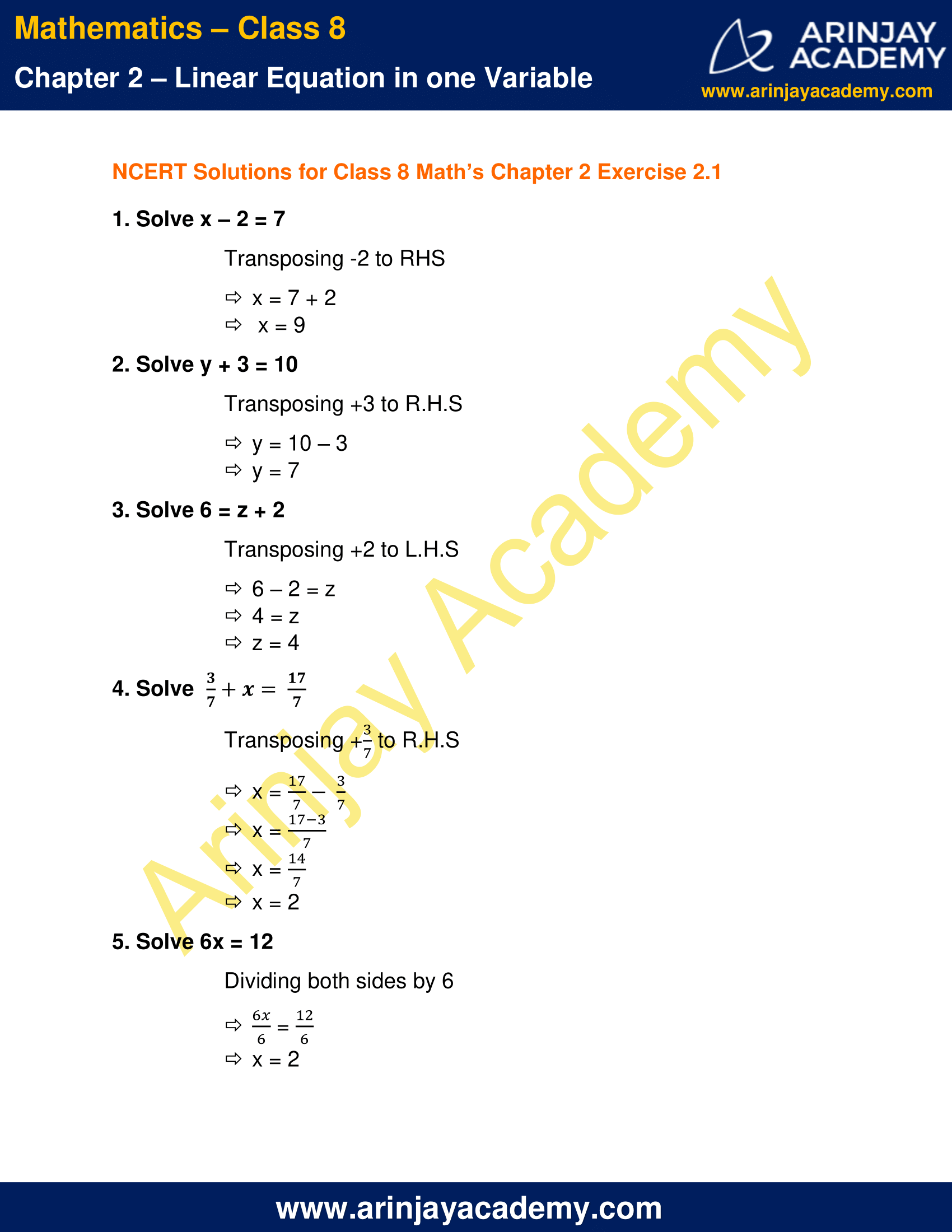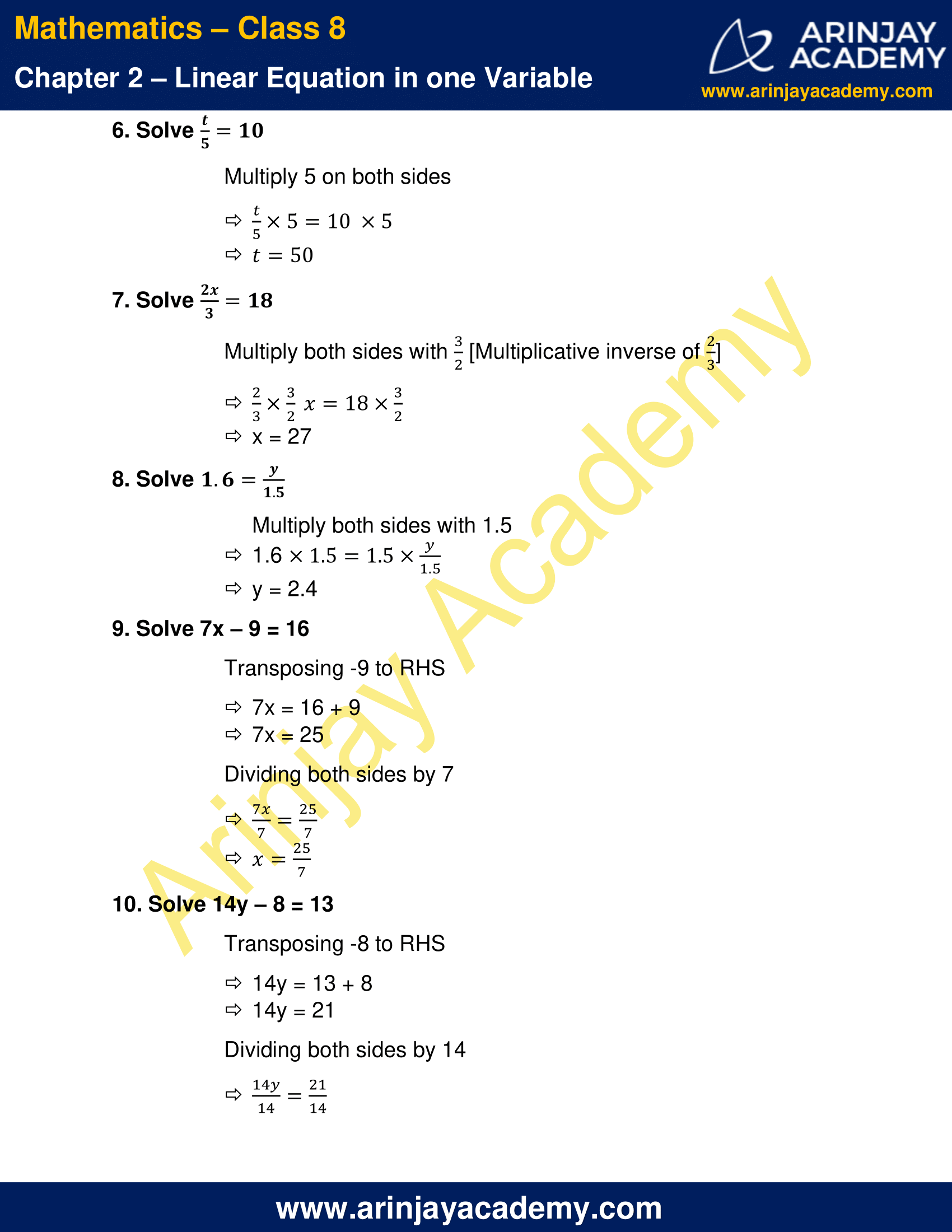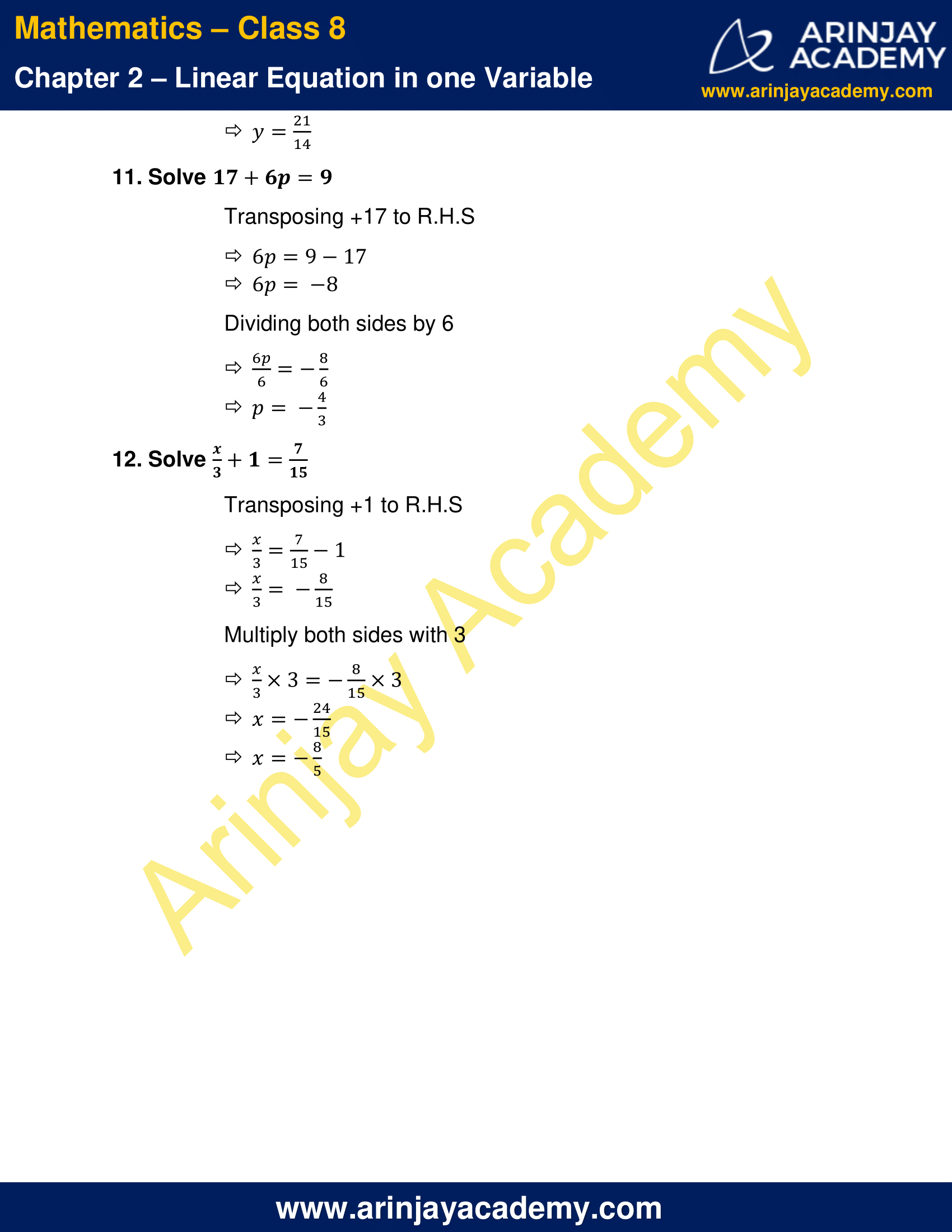# NCERT Solutions for Class 8 Maths Chapter 2 Exercise 2.1

NCERT Solutions for Class 8 Maths Chapter 2 Exercise 2.1 , has been designed by the NCERT to test the knowledge of the student on the following topics :

• Introduction
• Solving Equations which have Linear Expressions on one Side and Numbers on the other Side

### NCERT Solutions for Class 8 Maths Chapter 2 Exercise 2.1 – Linear Equation in one variableNCERT Solutions for Class 8 Maths Chapter 2 Exercise 2.1 – Linear Equation in one variable

1. Solve x – 2 = 7

Transposing -2 to RHS
x = 7 + 2
x = 9

2. Solve y + 3 = 10

Transposing +3 to R.H.S
y = 10 – 3
y = 7

3. Solve 6 = z + 2

Transposing +2 to L.H.S
6 – 2 = z
4 = z
z = 4

4. Solve (3/7) + x = (17/7)

Transposing + (3/7) to R.H.S
x = (17/7) – (3/7)
x = (17 – 3/7)
x = 14/7
x = 2

5. Solve 6x = 12

Dividing both sides by 6
6x/6 = 12/6
x = 2

6. Solve (t/5) = 10

Multiply 5 on both sides
(t/5) x 5 = 10 x 5
t = 50

7. Solve (2x/3) = 18

Multiply both sides with (3/2) [Multiplicative inverse of (2/3)]
(2x/3) x (3/2) = 18 x (3/2)
x = 27

8. Solve 1.6 = (y/1.5)

Multiply both sides with 1.5
1.6 x 1.5 = 1.5 x (y/1.5)
y = 2.4

9. Solve 7x – 9 = 16

Transposing -9 to RHS
7x = 16 + 9
7x = 25
Dividing both sides by 7
(7x/7) = (25/7)
x = (25/7)

10. Solve 14y – 8 = 13

Transposing -8 to RHS
14y = 13 + 8
14y = 21
Dividing both sides by 14
(14y/14) = (21/14)
y = 21/14

11. Solve 17 + 6p = 9

Transposing +17 to R.H.S
6p = 9 – 17
6p = -8
Dividing both sides by 6
(6p/6) = -(8/6)
p = -(4/3)

12. Solve (x/3) + 1 = (7/15)

Transposing +1 to R.H.S
(x/3) = (7/15) – 1
(x/3) = (-8/15)
Multiply both sides with 3
(x/3) x 3 = (-8/15) x 3
x = (-24/15)
x = (-8/5)

The next Exercise for NCERT Solutions for Class 8 Maths Chapter 2 Exercise 2.2 – Linear Equation in two Variables can be accessed by

Download NCERT Solutions for Class 8 Maths Chapter 2 Exercise 2.1 – Linear Equation in one Variable